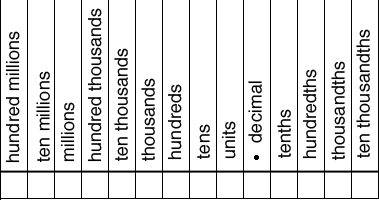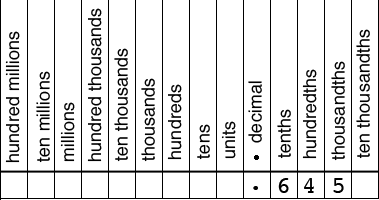SEARCH HOMEMath Central Quandaries & QueriesQuestion from Ruth: what does the 0 represent in .018 versus the decimal .180? As in, changing these to fractions? Thank you.Hi Ruth,

The 0 in the two decimal representations of the numbers have different place values. Below is a place value chart.Below I have written the decimal number 0.645 in the place value chart.Since 6 is in the tenths place it represents $\large \frac{6}{10}.$

Since 4 is in the hundredths place it represents $\large \frac{4}{100}.$

Since 5 is in the thousandths place it represents $\large \frac{5}{1000}.$

Thus

$0.645 = \frac{6}{10} + \frac{4}{100} + \frac{5}{1000}.$

Writing this with a common denominator I get

$0.645 = \frac{6 + 40 + 500}{1000} = \frac{645}{1000}.$

This fraction can be simplified.

In a similar fashion

$0.108 = \frac{1 + 0 + 800}{1000} = \frac{108}{1000}.$

This fraction can also be simplified.

I hope this helps,
Penny* Registered trade mark of Imperial Oil Limited. Used under license.Math Central is supported by the University of Regina and the Imperial Oil Foundation.